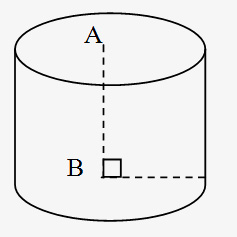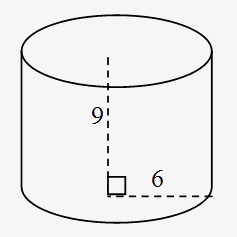# 3D Geometry: Mensuration- Right Prism & Cylinder

###### Understanding Three-Dimensional Geometry:
Most tests often include questions based on the knowledge of the geometries of 3-D objects such as cylinder, cone, cuboid, cube & sphere. The purpose of the article is to help you learn basics of 3-D geometry and encapsulate some of the important formulae and tricks.
The questions on Volume and Surface Area appear in all the competitive exams. Most of the students tend to avoid this topic considering it to be quite complex and calculative. This article would help you not only in memorizing the formulas, but also in understanding direct or indirect applications of these formulas. We strongly advice you go through each and every definition and formula given below to solve questions on Surface Area and Volume.
###### Surface area and Volume Formulas
Solids: Solids are three–dimensional objects, bound by one or more surfaces. Plane surfaces of a solid are called its faces. The lines of intersection of adjacent faces are called edges.
For any regular solid, Number of faces + Number of vertices = Number of edges + 2. This formula is called Euler’s formula.
Volume: Volume of a solid figure is the amount of space enclosed by its bounding surfaces. Volume is measured in cubic units.
###### Right Prism:
A prism is a solid, whose vertical faces are rectangular and whose bases are parallel polygons of equal area. A prism is said to be triangular prism, pentagonal prism, hexagonal prism, octagonal prism according to number of sides of the polygon that form the base. In a prism with a base of n sides, number of vertices = 2n, number of faces = n + 2.
Surface area formula of vertical faces of a prism = perimeter of base x height.
Total surface area of a prism = perimeter of base x height + 2 x area of base
Volume of a prism = area of base x height
1. The base of a prism is a triangle of sides 3, 4 and 5 m respectively. The height of the prism is 10 m. Find its volume.
1. 60 m3
2. 30 m3
3. 20 m3
4. 15 m3
5. 150 m3
Explanation: Volume of prism = Base Area x Height.
Base area = √s(s-a)(s-b)(s-a) where s = (3+4+5) / 2 = 6
Base area = √6 x 3 x 2 x 1 = 6m2
∴ Volume of prism = 6 x 10 = 60m3
Cylinder: The figure given below is right circular cylinder. The two bases are circles of the same size with centers A and B, respectively, and altitude (height) AB is perpendicular to the base.
The surface area of a right circular cylinder with a base of radius 'r' and height ‘h’ is equal to 2(πr2) + 2πrh (the sum of the areas of the two bases plus the area of the curved surface).
The volume of cylinder is equal to πr2h, that is (area of base) x (height).Volume of material of a hollow cylinder = π (R2 – r2) h, where R is outer radius and r is inner radius.
In the cylinder given below, surface area is equal to 2(36π) + 2π(6)(9) = 180π, and the volume is equal to 36π(9) = 324π.1. The height of a cylinder is 8 cm and the radius of the base is 3 cm. Find the area of the curved surface of the cylinder.
1. 120 sq cm
2. 140 sq cm
3. 150.72 sq cm
4. 180.2 sq cm
5. 200 sq cm
Explanation: The surface area = 2 π * r * h = 2 π * 3 * 8 = 150.72 sq. cm.
2. An open circular tank is dug 20 m deep. The earth is taken out and has been spread all round it, to form a circular embankment. The width of an Embankment is 2 m,& diameter of circular tank is 18m. Find the height of circular embankment.
1. 1.0 m
2. 1.5 m
3. 2.5 m
4. 1.97 m
Explanation: Volume of the Open Circular Tank = πr2h = π * [(18)/2]2 * 20 = π*9*9*20 m3.
Also, area of the earth dug out = π(9+2)2 - π(9)2
= π[(11+9)(11-9)] = π(20)(2) = 40πm2
∴ Height of circular embankment = (= π*9*9*20 )/( 40π ) = 40.5m.